# Surds

## Introduction

Surds are numbers left in root form (√) to express its exact value. It has an infinite number of non-recurring decimals. Therefore, surds are irrational numbers.

There are certain rules that we follow to simplify an expression involving surds. Rationalising the denominator is one way to simplify these expressions. It is done by eliminating the surd in the denominator. This is shown in Rules 3, 5 and 6.

It can often be necessary to find the largest perfect square factor in order to simplify surds. The largest perfect square factor is found by looking at any possible factors of the number that is being square rooted. Lets say that you are looking at the square root of 242. Can you simplify this? Well, 2 x 121 is 242 and we can take the square root of 121 without leaving a surd (because we get 11). Since we cannot take the square root of a larger number that can be multiplied by another to give 242 then we say that 121 is the largest perfect square factor.

## Six Rules of Surds

 Rule 1: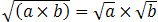An Example:

Simplify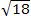:

Since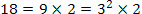, as 9 is the largest perfect square factor of 18.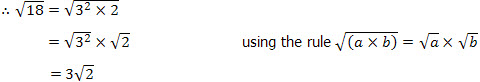Rule 2: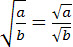An Example:

 Simplify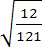: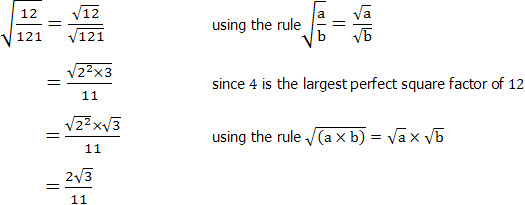Rule 3: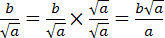By multiplying both the numberator and denominator by the denominator you can rationalise the denominator.

An Example:

 rationalise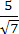: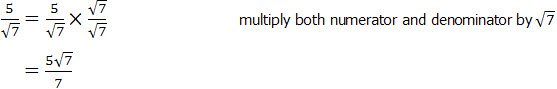Rule 4: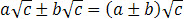An Example:

 Simplify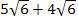: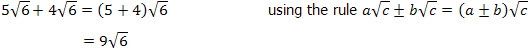Rule 5: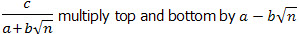Following this rule enables you to rationalise the denominator.

An Example:

 Rationalise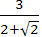: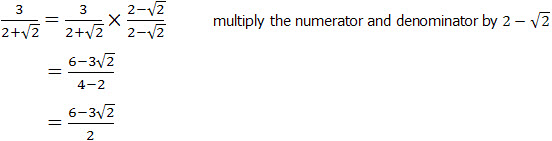Rule 6: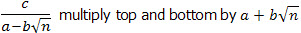Following this rule enables you to rationalise the denominator.

An Example:

 Rationalise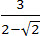: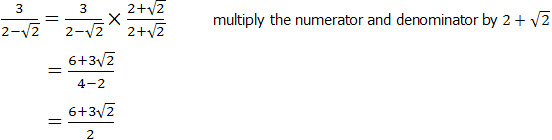You have now learnt the important rules of surds.

Go to the next page to start practicing what you have learnt.